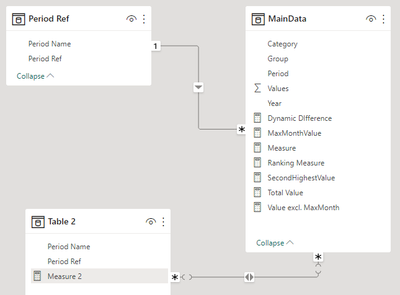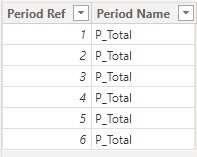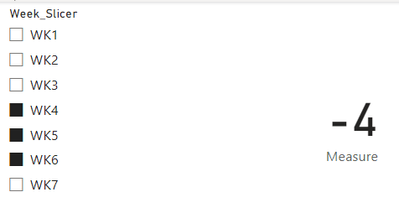cancel
Showing results for
Did you mean:

Fabric is Generally Available. Browse Fabric Presentations. Work towards your Fabric certification with the Cloud Skills Challenge.Resolver I

## Comparing Values with Offset?

Hey,

I'd like to create a measure that can help me find the difference between 2 values but not sure of the best way to do it.

I have a week slicer that goes from 1-6 and I'd like to create a measure that compares the latest value so like Week 6 with the other largest selected slicer value.

So if slicer had WK6, WK2, WK4 selected: Comparison would be WK6-WK4

If slicer had WK4, WK2, WK1 selected: Comparison would be WK4-WK2

If slicer had WK5, WK4, WK3 selected: Comparison would be WK5-WK4

How can I get this consistent everytime?

1 ACCEPTED SOLUTIONCommunity Support

Hi  @TotunG ,

Here are the steps you can follow：

1. You reposition the two columns of the Period Ref table, with [Period Ref] first and [Period Name] last.2. Create calculated column.

``````Table 2 =
var _table1=
SUMMARIZE(
'Table','Table'[Period Ref],
"Period Name",
"P_Total")
return
UNION(
'Table',_table1)``````3. Combine the relationship between two tables.4. Create measure.

``````Flag=
IF(
MAX('Table 2'[Period Ref]) in SELECTCOLUMNS('MainData',"1",[Period]) && MAX('Table 2'[Period Name]) <> "P_Total",
[Total Value],[Dynamic DIfference])``````

5. Result:Best Regards,

Liu Yang

If this post helps, then please consider Accept it as the solution to help the other members find it more quickly

8 REPLIES 8Community Support

Hi  @TotunG ,

``````Table 2 =
var _table1=
SUMMARIZE(
'Table','Table'[Period Ref],
"Period Name",
"P_Total")
return
UNION(
'Table',_table1)``````

This allows the new table to be appended to the previous table using the Union() formula, forming

UNION function (DAX) - DAX | Microsoft Learn

SUMMARIZE function (DAX) - DAX | Microsoft LearnThis will add a column of p_Total when it is put into the matrixThis adds a column of p_Total when it is put into the matrix

``Flag=IF(    MAX('Table 2'[Period Ref]) in SELECTCOLUMNS('MainData',"1",[Period]) && MAX('Table 2'[Period Name]) <> "P_Total",    [Total Value],[Dynamic DIfference])``

SELECTCOLUMNS('MainData',"1",[Period])：

Returns a table that contains the columns of [Period]

This is used to determine whether 'Table 2[Period ref] exists in the [Period] of Main Data Table and the [Period Name] of Table2 is not the character "p_Total", if everything matches, then it is [Total Value], otherwise the result is [Dynamic DIfference])

IF function (DAX) - DAX | Microsoft Learn

SELECTCOLUMNS function (DAX) - DAX | Microsoft Learn

Best Regards,

Liu Yang

If this post helps, then please consider Accept it as the solution to help the other members find it more quickly.Community Support

Hi  @TotunG ,

Here are the steps you can follow：

1. You reposition the two columns of the Period Ref table, with [Period Ref] first and [Period Name] last.2. Create calculated column.

``````Table 2 =
var _table1=
SUMMARIZE(
'Table','Table'[Period Ref],
"Period Name",
"P_Total")
return
UNION(
'Table',_table1)``````3. Combine the relationship between two tables.4. Create measure.

``````Flag=
IF(
MAX('Table 2'[Period Ref]) in SELECTCOLUMNS('MainData',"1",[Period]) && MAX('Table 2'[Period Name]) <> "P_Total",
[Total Value],[Dynamic DIfference])``````

5. Result:Best Regards,

Liu Yang

If this post helps, then please consider Accept it as the solution to help the other members find it more quicklyResolver I

Seems like it might work - will try this out. Do you mind briefly breaking down the steps in this and why you did them? No worries if not as appreciate your help regardless.Community Support

Hi  @TotunG ,

It's not clear what your table structure is, you can try placing the formula in a PBIX file to see if that works.

If the results are not what you expected, Can you share sample data and sample output in table format? Or a sample pbix after removing sensitive data. We can better understand the problem and help you.

Best Regards,

Liu Yang

If this post helps, then please consider Accept it as the solution to help the other members find it more quicklyResolver I

Okay sample file is attached with explaination (also a photo if needed). There is a relationship between 2 tables with columns Period and Period Name so that user can use Period Name to filter MainData periods:Community Support

Hi  @TotunG ,

I created some data:Here are the steps you can follow：

1. Create measure.

``````Measure =
var _select=
SELECTCOLUMNS('Table',"1",[Week_Slicer])
var _maxx=
MAXX(
FILTER(ALL('Table'),'Table'[Week_Slicer] in _select),[Week])
var _secondlargest=
MAXX(
FILTER(ALL('Table'),'Table'[Week_Slicer] in _select&&'Table'[Week]<_maxx),[Week])
return
SUMX(
FILTER(ALL('Table'),'Table'[Week]=_maxx),[Value])
-
SUMX(
FILTER(ALL('Table'),'Table'[Week]=_secondlargest),[Value])``````

2. Result:Best Regards,

Liu Yang

If this post helps, then please consider Accept it as the solution to help the other members find it more quicklyResolver I

Thank you - would this still work if Week table is different from the Data table but connected with a relationship and the value being filtered is a measure?Super User

should be possible, even without offset.  Ideally you would have numeric week numbers though, otherwise you run into trouble comparing WK10 with WK6 (for example)Announcements#### Power BI Monthly Update - November 2023

Check out the November 2023 Power BI update to learn about new features.#### Fabric Community News unified experience

Read the latest Fabric Community announcements, including updates on Power BI, Synapse, Data Factory and Data Activator.#### The largest Power BI and Fabric virtual conference

130+ sessions, 130+ speakers, Product managers, MVPs, and experts. All about Power BI and Fabric. Attend online or watch the recordings.Top Solution Authors
Top Kudoed Authors
Users online (4,309)